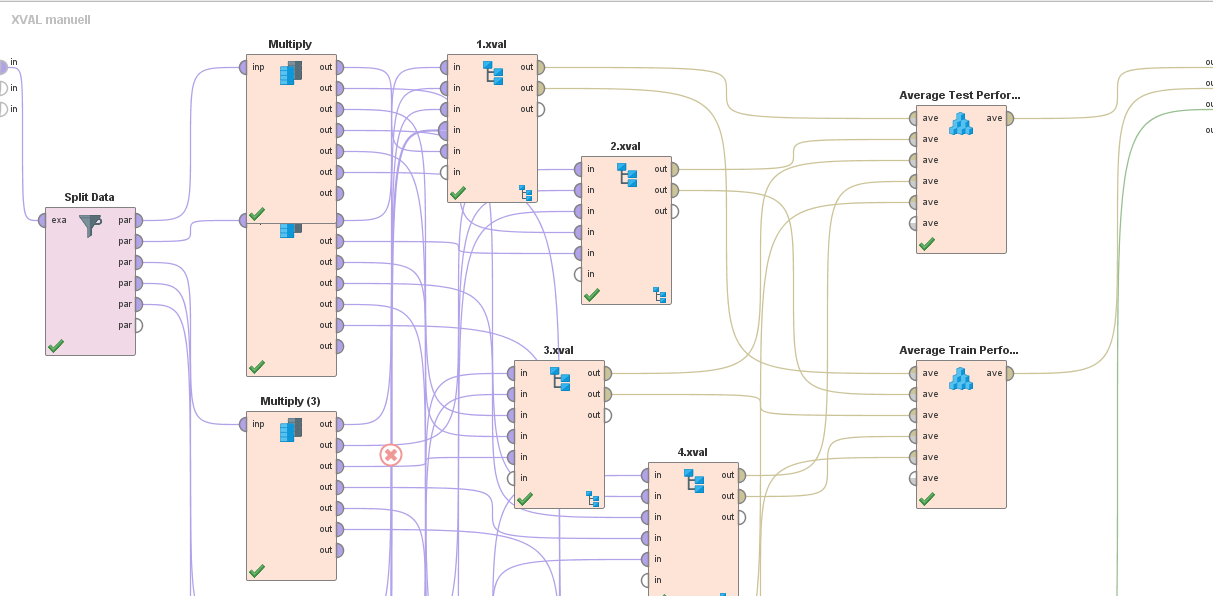# Combine performance vectors into one average pv, and log it?

Member Posts: 344Unicorn
edited November 2018 in Help

hi,

I tried to combine several performance vectors into one, and get the average performance vector, thats possible with the average operator...

however when I try to log the avg perf vector, there is no way to do it?? I cannot generate attribute out of it, and it apparently has no field "performance" from the average operator that could be logged... I hope thats a bug, not a feature...

I also cannot use extract log operator, as it expects some named attribute..

here is a picture of what I was trying to do, basically I did a manual 5-fold X-Validation and want to average the train and test-performances of each run into one:

I could just connect the output port of the average to the results set, however, I want to log it as there are multiple runs when I do this in a grid optimization with different parameters, and otherwise, I only get the last output of the average perf vector...average several performances into one

Tagged:

• Administrator, Moderator, Employee, RapidMiner Certified Analyst, RapidMiner Certified Expert, University Professor Posts: 2,238RM Data Scientist

wow,

you might want to use a loop? Have a look at this process:

`<?xml version="1.0" encoding="UTF-8"?><process version="7.2.001">  <context>    <input/>    <output/>    <macros/>  </context>  <operator activated="true" class="process" compatibility="7.2.001" expanded="true" name="Process">    <process expanded="true">      <operator activated="true" class="retrieve" compatibility="7.2.001" expanded="true" height="68" name="Retrieve Sonar" width="90" x="45" y="85">        <parameter key="repository_entry" value="//Samples/data/Sonar"/>      </operator>      <operator activated="true" class="extract_macro" compatibility="7.2.001" expanded="true" height="68" name="Extract Macro" width="90" x="179" y="85">        <parameter key="macro" value="numberOfExamples"/>        <list key="additional_macros"/>      </operator>      <operator activated="true" class="generate_id" compatibility="7.2.001" expanded="true" height="82" name="Generate ID" width="90" x="313" y="85"/>      <operator activated="true" class="loop" compatibility="7.2.001" expanded="true" height="103" name="Loop" width="90" x="514" y="85">        <parameter key="set_iteration_macro" value="true"/>        <parameter key="iterations" value="5"/>        <process expanded="true">          <operator activated="true" class="generate_macro" compatibility="7.2.001" expanded="true" height="82" name="Generate Macro" width="90" x="45" y="34">            <list key="function_descriptions">              <parameter key="max" value="round(&#10;&#10;eval(%{numberOfExamples}) - (eval(%{iteration})-1)/5*eval(%{numberOfExamples})&#10;&#10;)-1"/>              <parameter key="min" value="round(&#10;&#10;eval(%{numberOfExamples}) - eval(%{iteration})/5*eval(%{numberOfExamples})&#10;&#10;)"/>            </list>          </operator>          <operator activated="true" class="filter_example_range" compatibility="7.2.001" expanded="true" height="82" name="Filter Example Range" width="90" x="179" y="34">            <parameter key="first_example" value="%{min}"/>            <parameter key="last_example" value="%{max}"/>          </operator>          <operator activated="true" class="x_validation" compatibility="5.0.000" expanded="true" height="124" name="Validation" width="90" x="447" y="34">            <parameter key="sampling_type" value="2"/>            <process expanded="true">              <operator activated="true" class="parallel_decision_tree" compatibility="7.2.001" expanded="true" height="82" name="Decision Tree" width="90" x="45" y="30"/>              <connect from_port="training" to_op="Decision Tree" to_port="training set"/>              <connect from_op="Decision Tree" from_port="model" to_port="model"/>              <portSpacing port="source_training" spacing="0"/>              <portSpacing port="sink_model" spacing="0"/>              <portSpacing port="sink_through 1" spacing="0"/>            </process>            <process expanded="true">              <operator activated="true" class="apply_model" compatibility="5.0.000" expanded="true" height="82" name="Apply Model" width="90" x="45" y="30">                <list key="application_parameters"/>              </operator>              <operator activated="true" class="performance_binominal_classification" compatibility="7.2.001" expanded="true" height="82" name="Performance (2)" width="90" x="179" y="85"/>              <connect from_port="model" to_op="Apply Model" to_port="model"/>              <connect from_port="test set" to_op="Apply Model" to_port="unlabelled data"/>              <connect from_op="Apply Model" from_port="labelled data" to_op="Performance (2)" to_port="labelled data"/>              <connect from_op="Performance (2)" from_port="performance" to_port="averagable 1"/>              <portSpacing port="source_model" spacing="0"/>              <portSpacing port="source_test set" spacing="0"/>              <portSpacing port="source_through 1" spacing="0"/>              <portSpacing port="sink_averagable 1" spacing="0"/>              <portSpacing port="sink_averagable 2" spacing="0"/>            </process>            <description align="center" color="transparent" colored="false" width="126">A cross-validation evaluating a decision tree model.</description>          </operator>          <operator activated="true" class="performance_to_data" compatibility="7.2.001" expanded="true" height="82" name="Performance to Data" width="90" x="581" y="85"/>          <connect from_port="input 1" to_op="Generate Macro" to_port="through 1"/>          <connect from_op="Generate Macro" from_port="through 1" to_op="Filter Example Range" to_port="example set input"/>          <connect from_op="Filter Example Range" from_port="example set output" to_op="Validation" to_port="training"/>          <connect from_op="Validation" from_port="model" to_port="output 1"/>          <connect from_op="Validation" from_port="averagable 1" to_op="Performance to Data" to_port="performance vector"/>          <connect from_op="Performance to Data" from_port="example set" to_port="output 2"/>          <portSpacing port="source_input 1" spacing="0"/>          <portSpacing port="source_input 2" spacing="0"/>          <portSpacing port="sink_output 1" spacing="0"/>          <portSpacing port="sink_output 2" spacing="0"/>          <portSpacing port="sink_output 3" spacing="0"/>        </process>      </operator>      <operator activated="true" class="append" compatibility="7.2.001" expanded="true" height="82" name="Append" width="90" x="648" y="136"/>      <operator activated="true" class="aggregate" compatibility="7.2.001" expanded="true" height="82" name="Aggregate" width="90" x="782" y="136">        <list key="aggregation_attributes">          <parameter key="Value" value="average"/>        </list>      </operator>      <connect from_op="Retrieve Sonar" from_port="output" to_op="Extract Macro" to_port="example set"/>      <connect from_op="Extract Macro" from_port="example set" to_op="Generate ID" to_port="example set input"/>      <connect from_op="Generate ID" from_port="example set output" to_op="Loop" to_port="input 1"/>      <connect from_op="Loop" from_port="output 1" to_port="result 1"/>      <connect from_op="Loop" from_port="output 2" to_op="Append" to_port="example set 1"/>      <connect from_op="Append" from_port="merged set" to_op="Aggregate" to_port="example set input"/>      <connect from_op="Aggregate" from_port="example set output" to_port="result 2"/>      <portSpacing port="source_input 1" spacing="0"/>      <portSpacing port="sink_result 1" spacing="0"/>      <portSpacing port="sink_result 2" spacing="0"/>      <portSpacing port="sink_result 3" spacing="0"/>    </process>  </operator></process>`
- Head of Data Science Services at RapidMiner -
Dortmund, Germany
• Member Posts: 344Unicorn

hm ich bekomms nicht hin, keine Ahnung wie ich eine Loop drumherum bauen soll, und es gibt keinen einfacheren Weg mehrere Performance Werte zu aggregieren und zu extrahieren?

• Administrator, Moderator, Employee, RapidMiner Certified Analyst, RapidMiner Certified Expert, University Professor Posts: 2,238RM Data Scientist

did you check my process? It should do very similar things to what you did in the screenshot

- Head of Data Science Services at RapidMiner -
Dortmund, Germany
• Administrator, Moderator, Employee, RapidMiner Certified Analyst, RapidMiner Certified Expert, Community Manager, RMResearcher, Member, University Professor Posts: 1,723RM Founder

Hi,

The trick would be to first use "Performance to Data" and then "Extract Log".

BTW: you get the 1st price for the annual "most crossing connections in a RapidMiner process" competition :smileyhappy:  -  very impressive!

Best,

Ingo